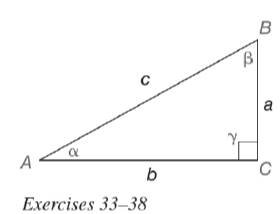Chapter 11.3, Problem 36EElementary Geometry For College St...

7th Edition
Alexander + 2 others
ISBN: 9781337614085

Solutions

Chapter
SectionElementary Geometry For College St...

7th Edition
Alexander + 2 others
ISBN: 9781337614085
Textbook Problem

In Exercises 33 to 38, we expand the list of trigonometric identities. As you may recall, an identity is a statement that is true for all permissible choices of the variable (see page 510.)a) For α ≠ 0 ∘ , prove the identity csc α = 1 sin α . b) Use your calculator to determine csc 12.3 ∘ .To determine

(a)

To prove:

For α0, the identity cscα=1sinα.

Explanation

Given:

For α0, cscα=1sinα.

Definition used:

The trigonometric ratios:

 sinα=oppositehypotenuse cosecα=hypotenuseopposite

Calculation:

Consider the angle α.

From the figure, the length of the opposite leg is a, the length of the adjacent leg is b and the hypotenuse is c.

sinα=ac

cscα=ca   ...(1)

Consider 1sinα.

To determine

(b)

To find:

The value of csc12.3 using the calculator.

Still sussing out bartleby?

Check out a sample textbook solution.

See a sample solution

The Solution to Your Study Problems

Bartleby provides explanations to thousands of textbook problems written by our experts, many with advanced degrees!

Get Started

Place the following set of n = 20 scores in a frequency distribution table.

Essentials of Statistics for The Behavioral Sciences (MindTap Course List)

Evaluate the integral. 4. 0/6tsin2tdt

Single Variable Calculus: Early Transcendentals

limx0+(1+x)1/x= a) e b) e c) 1e d) 1e

Study Guide for Stewart's Single Variable Calculus: Early Transcendentals, 8th

The unit vector in the direction of is:

Study Guide for Stewart's Multivariable Calculus, 8th